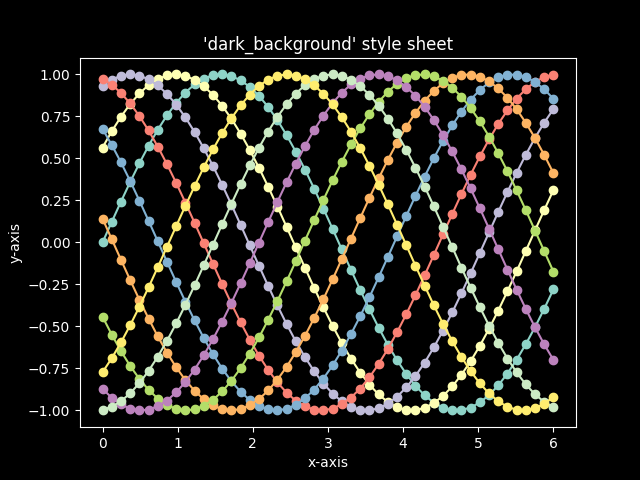# Dark background style sheet¶

This example demonstrates the “dark_background” style, which uses white for elements that are typically black (text, borders, etc). Note that not all plot elements default to colors defined by an rc parameter.```import numpy as np
import matplotlib.pyplot as plt

plt.style.use('dark_background')

fig, ax = plt.subplots()

L = 6
x = np.linspace(0, L)
ncolors = len(plt.rcParams['axes.prop_cycle'])
shift = np.linspace(0, L, ncolors, endpoint=False)
for s in shift:
ax.plot(x, np.sin(x + s), 'o-')
ax.set_xlabel('x-axis')
ax.set_ylabel('y-axis')
ax.set_title("'dark_background' style sheet")

plt.show()
```

Keywords: matplotlib code example, codex, python plot, pyplot Gallery generated by Sphinx-Gallery